You are currently offline. Some features of the site may not work correctly.

# Cycle space

In graph theory, a branch of mathematics, the (binary) cycle space of an undirected graph is the set of its Eulerian subgraphs. This set of subgraphs… Expand
Wikipedia

## Papers overview

Semantic Scholar uses AI to extract papers important to this topic.
2016
2016
• Discret. Appl. Math.
• 2016
• Corpus ID: 36479423
Let G be a undirected graph without loops and multiple edges. By ź ( G ) , ź ( G ) and p ( G ) we respectively denote the nullity… Expand
Is this relevant?
2014
2014
• SIAM J. Discret. Math.
• 2014
• Corpus ID: 5458395
The competition graph of an acyclic directed graph $D$ is the undirected graph on the same vertex set as $D$ in which two… Expand
•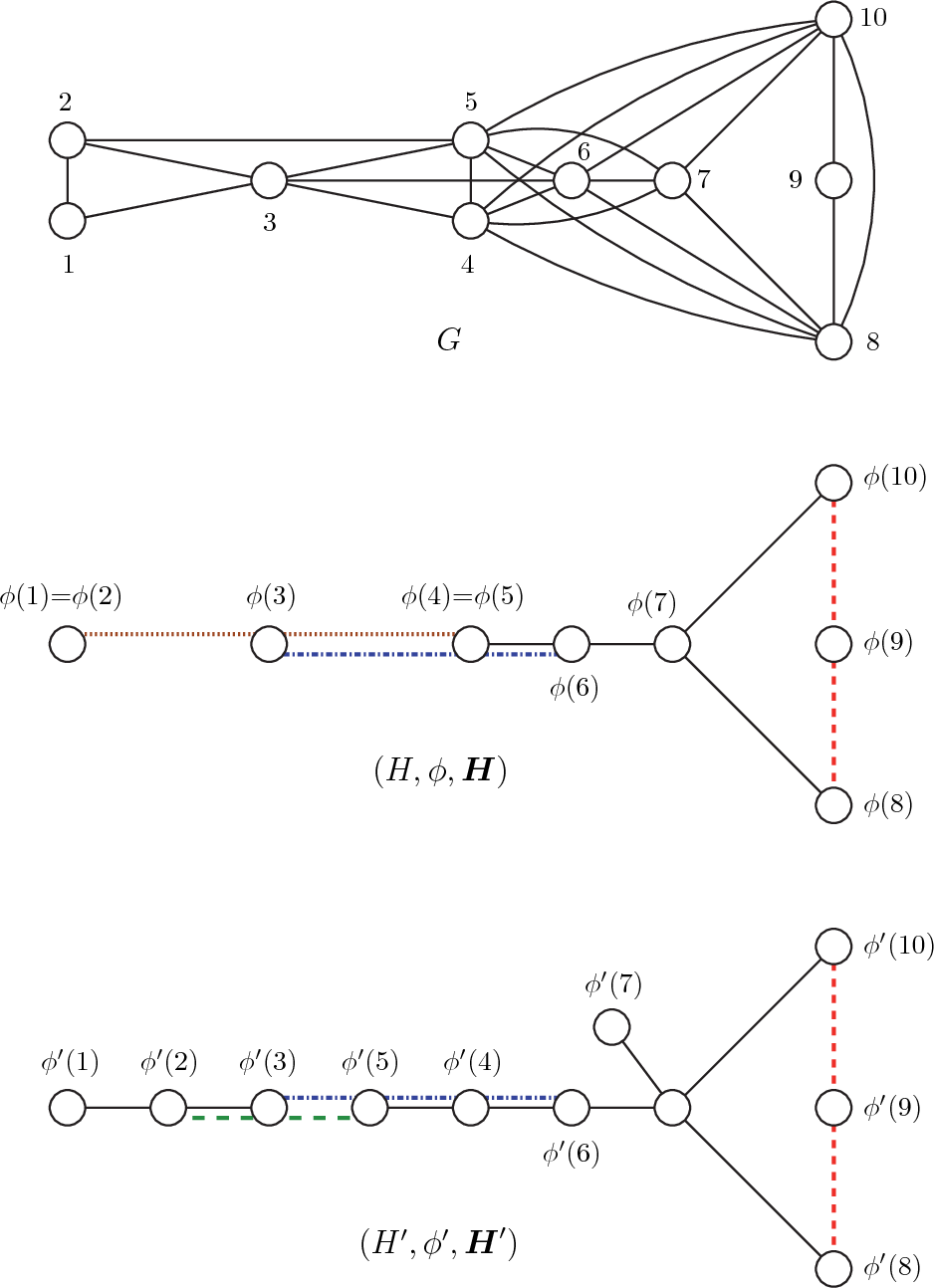•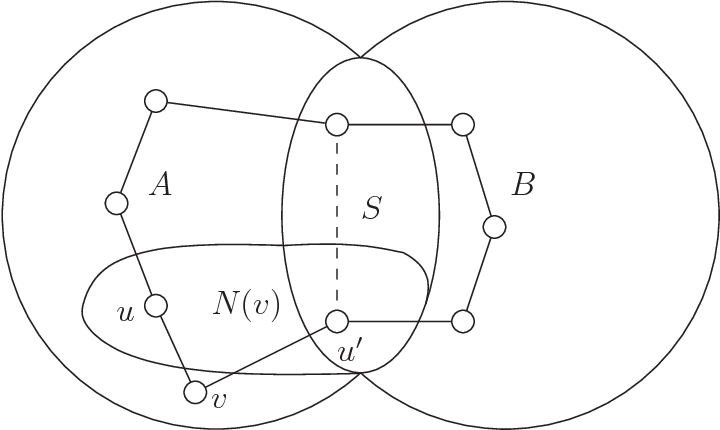Is this relevant?
2013
2013
• 2013
• Corpus ID: 55984025
Delayed interactions are a common property of coupled natural systems and therefore arise in a variety of different applications… Expand
•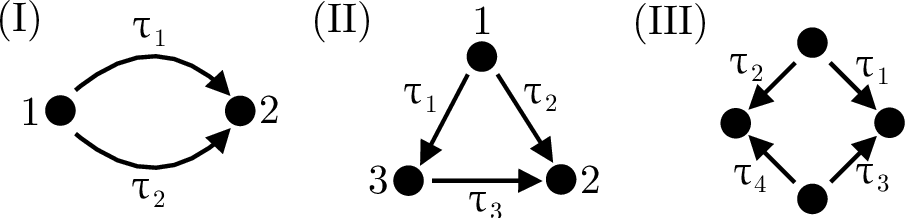•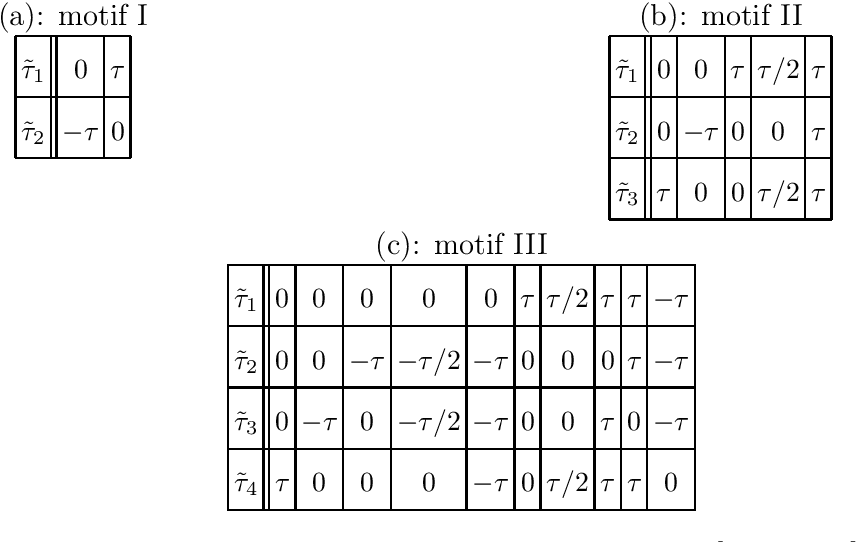•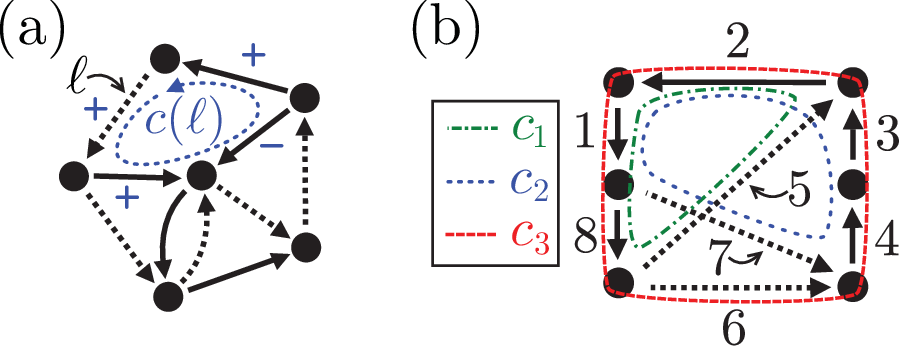•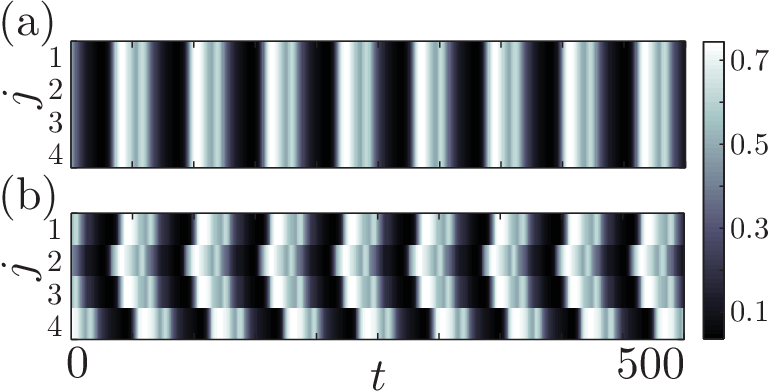•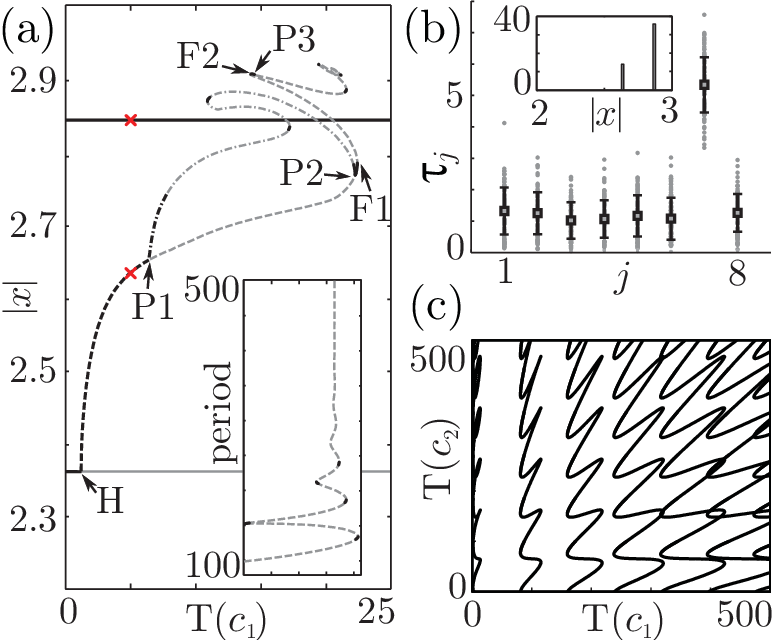Is this relevant?
2012
2012
• 2012
• Corpus ID: 15270676
We show that the series of all walks between any two vertices of any (possibly weighted) directed graph $\mathcal{G}$ is given by… Expand
Is this relevant?
2010
2010
• ACC
• 2010
• Corpus ID: 121331314
This paper addresses the problem of estimating the states of a group of agents from noisy measurements of pairwise differences… Expand
Is this relevant?
2008
2008
• Journal of Graph Theory
• 2008
• Corpus ID: 6867759
We develop a general model of edge spaces in order to generalize, unify, and simplify previous work on cycle spaces of infinite… Expand
Is this relevant?
2005
2005
• Inf. Process. Lett.
• 2005
• Corpus ID: 5203238
We consider the problem of computing a minimum cycle basis of a directed graph with m arcs and n nodes. We adapt the greedy… Expand
•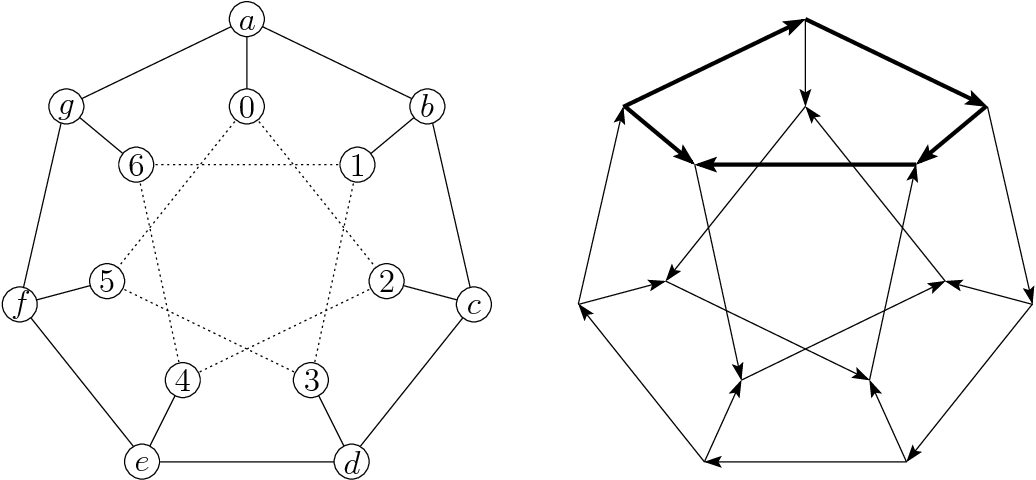•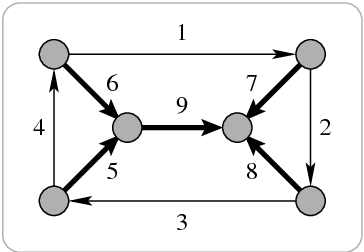Is this relevant?
2001
2001
An undirected biconnected graph G with non negative weights on the edges is given. In the cycle space associated with G, a… Expand
Is this relevant?
1982
1982
• Discret. Math.
• 1982
• Corpus ID: 9393991
We prove a theorem implying the conjecture of Woodall  that, given any k independent edges in a (k+1)-connected graph, there… Expand
Is this relevant?
Highly Cited
1979
Highly Cited
1979
• M. Syslo
• Discret. Math.
• 1979
• Corpus ID: 5082937
Abstract The paper presents several characterizations of outerplanar graphs, some of them are counterparts of the well-known… Expand
Is this relevant?